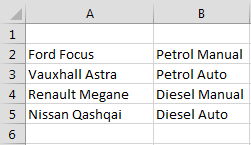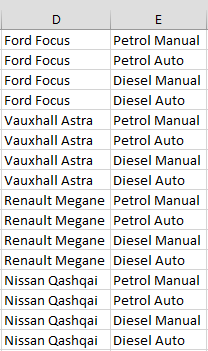# Cross Join Columns in Excel

As part of a project I’m working on I need to create a cross join of users against symbols; the idea being that any of the given users might look at any of the given symbols. I have a list of users and a list of symbols which I essentially need a cross-join on.

For this example I’ll use cars and engine/transmission variants.From these two lists I need to quickly create all possible combinations;I can’t find any function that will do what I was so I’ve created a VBA function that will do it;

``````Function CrossJoin(r1 As Range, r2 As Range) As Variant
Dim size As Integer
Dim arr As Variant
Dim offset As Integer
size = r1.Rows.Count * r2.Rows.Count
ReDim arr(0 To size, 0 To 1)
offset = 0

For i = 1 To r1.Rows.Count
For j = 1 To r2.Rows.Count
arr(offset, 0) = r1.Cells(i, 1).Value2
arr(offset, 1) = r2.Cells(j, 1).Value2
offset = offset + 1
Next j
Next i

CrossJoin = arr
End Function
``````

To use the function select and empty cell and insert `=CROSSJOIN(A1:A4, B1:B4)` (adjusting the input range as required) then when the function has evaluated extend the range to the size of the expected cross join. (in this case 16x2).

To calculate the array function hit `F2` then press `Ctrl+Shift+Enter` to calculate the array.